# Fig 16 1 p 488 Fig 16 2

• Slides: 38
Download presentation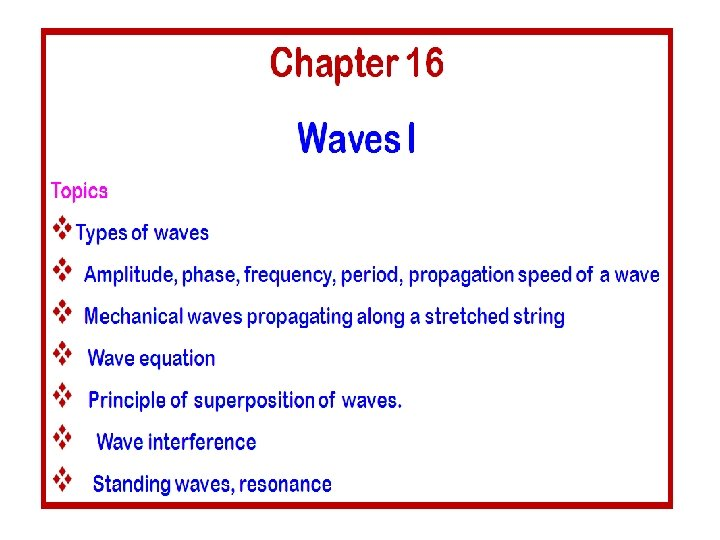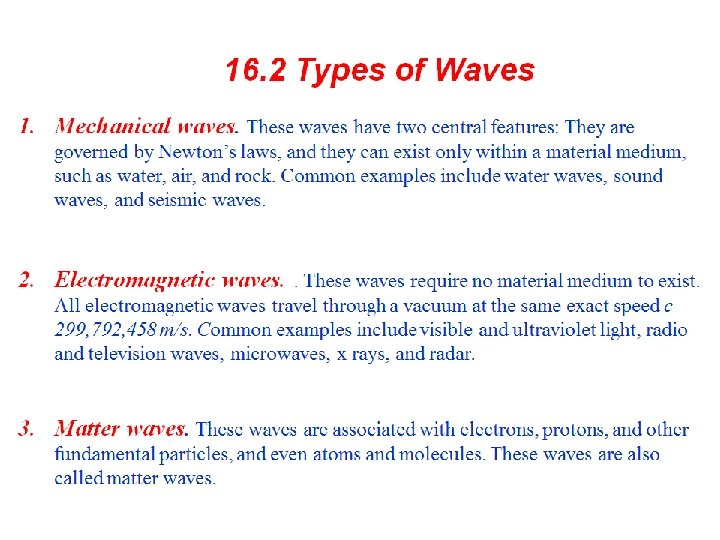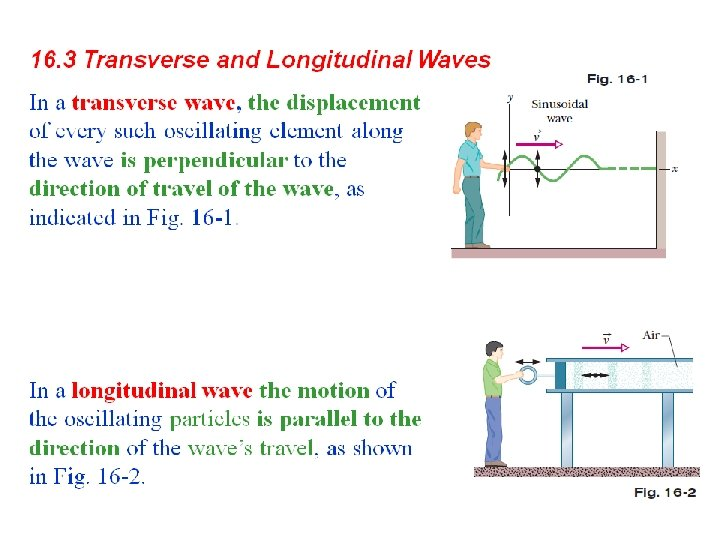Fig. 16. 1, p. 488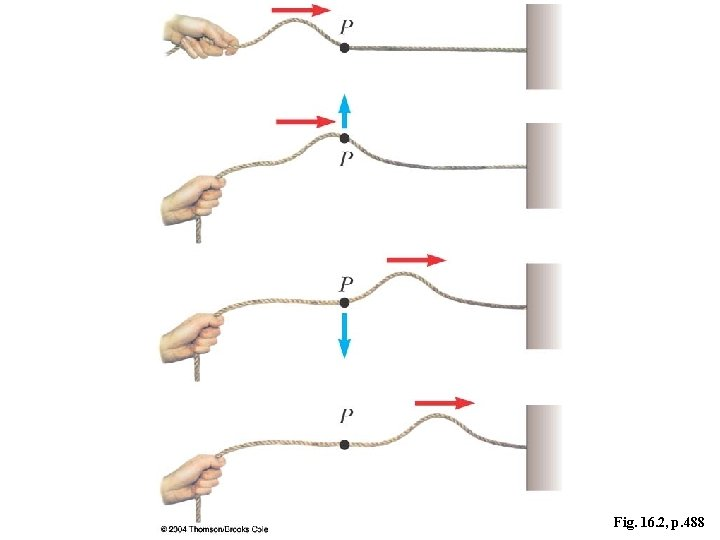Fig. 16. 2, p. 488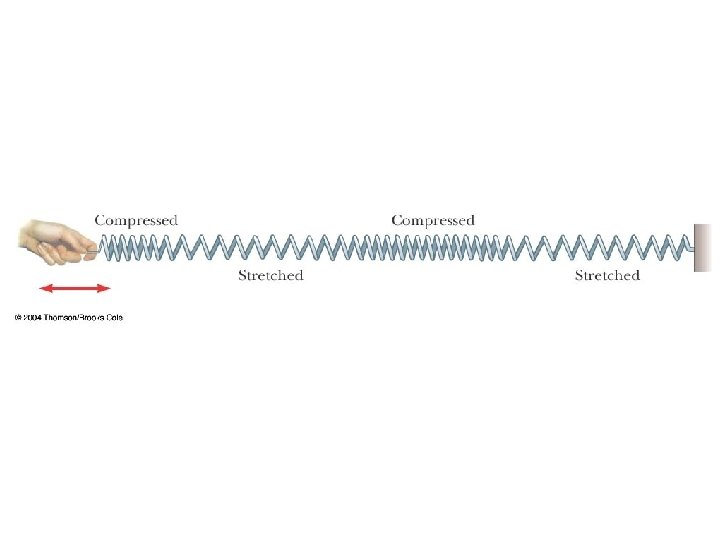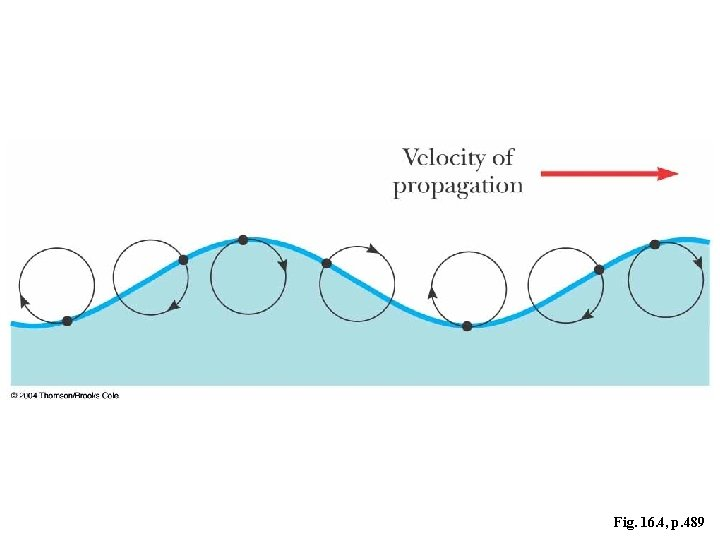Fig. 16. 4, p. 489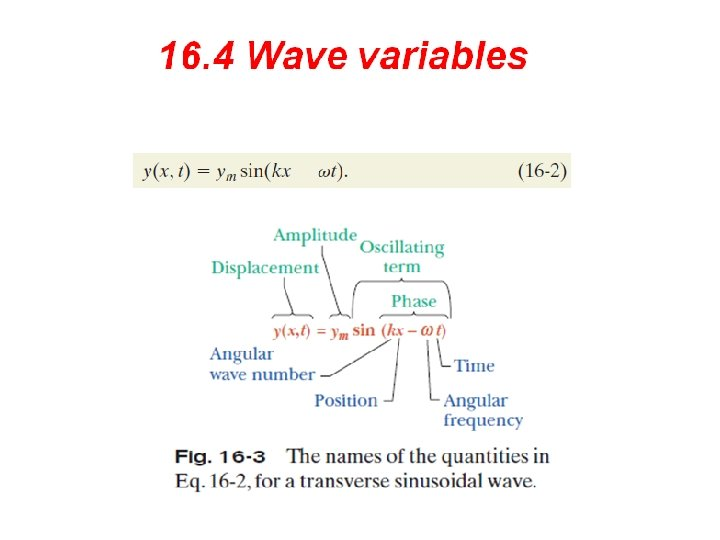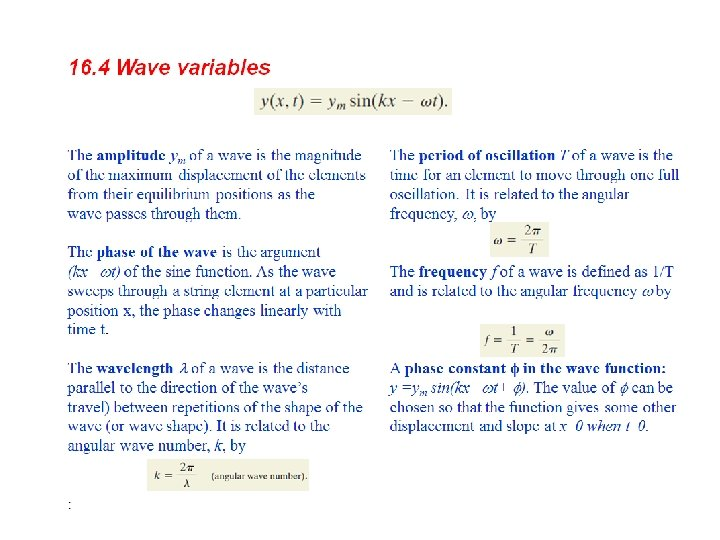Fig. 16. 8, p. 492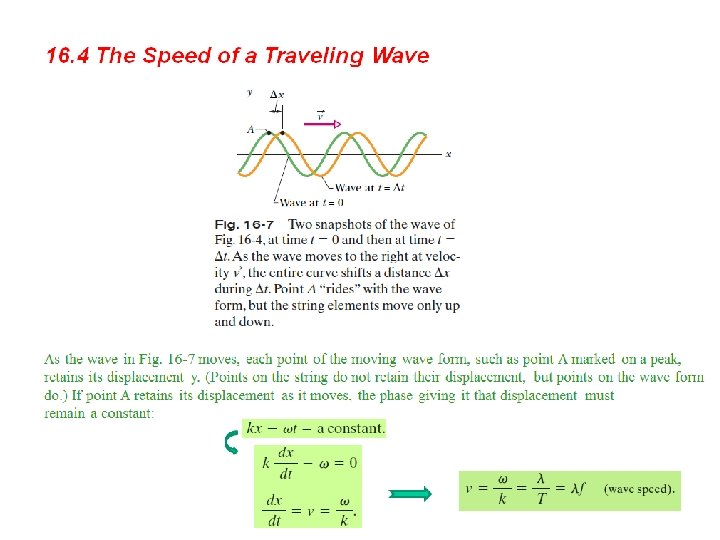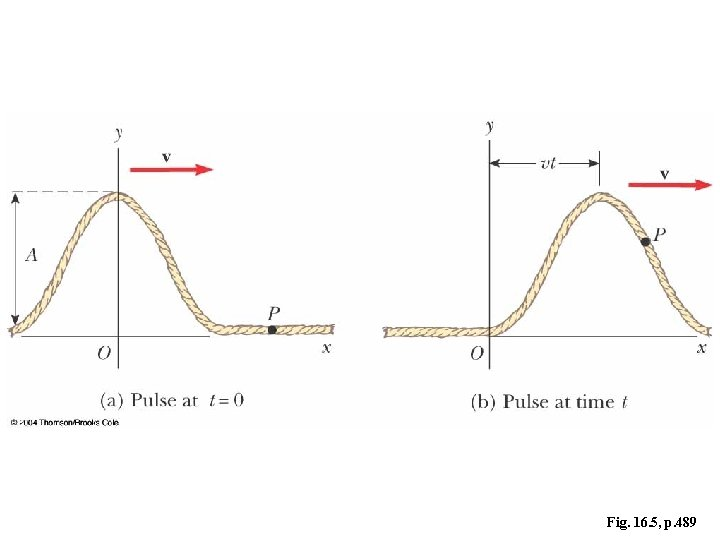Fig. 16. 5, p. 489Fig. 16. 6, p. 491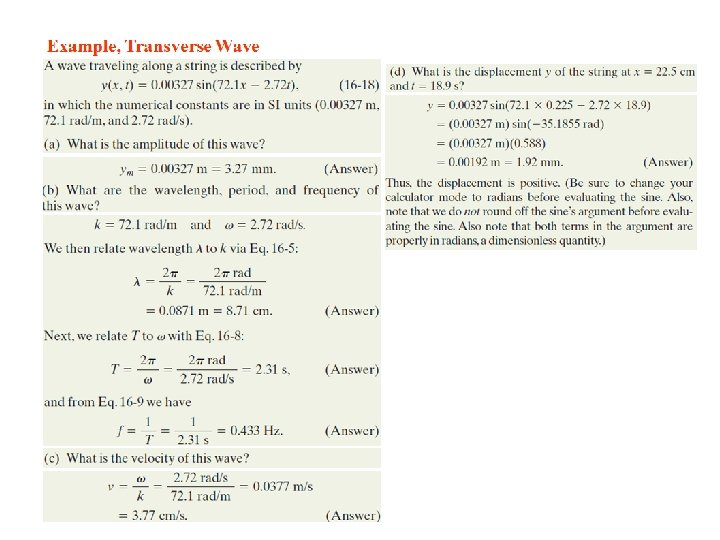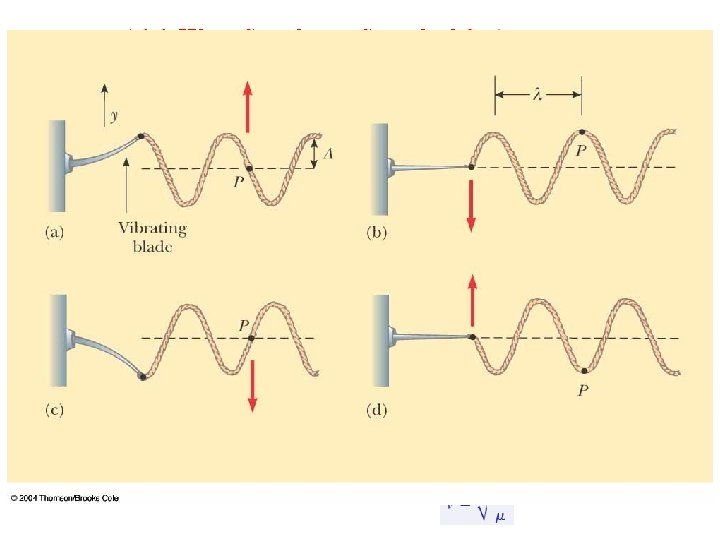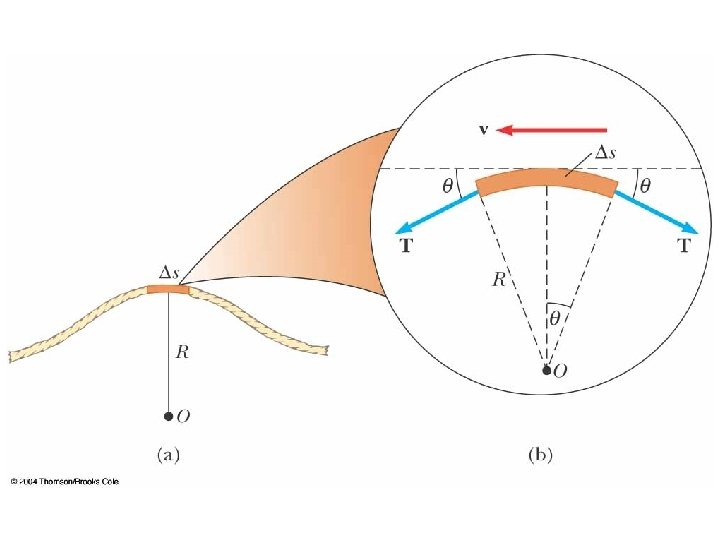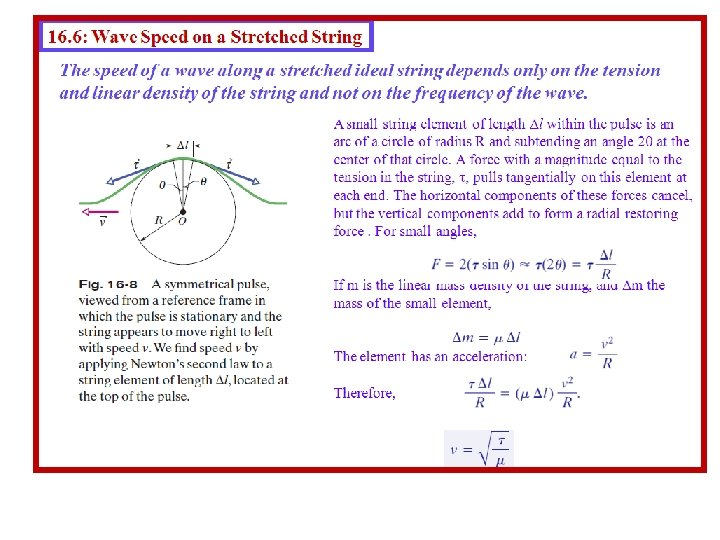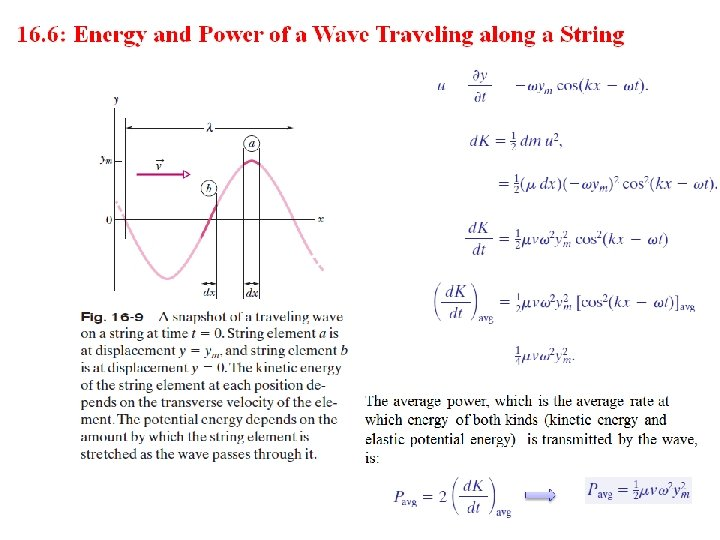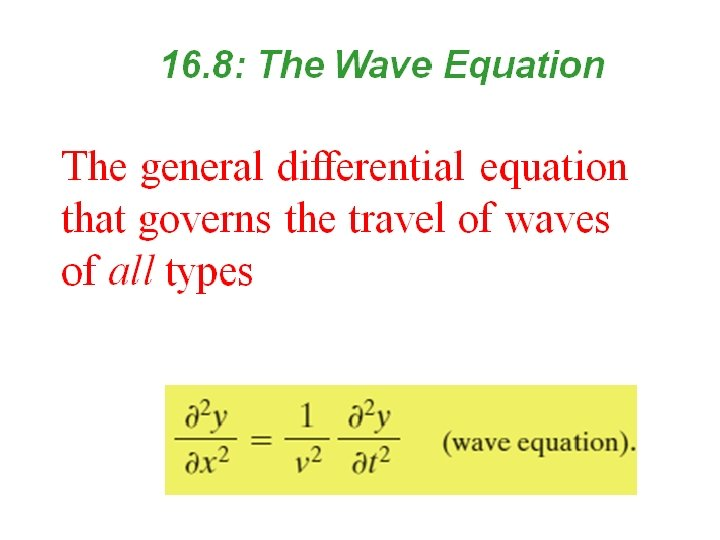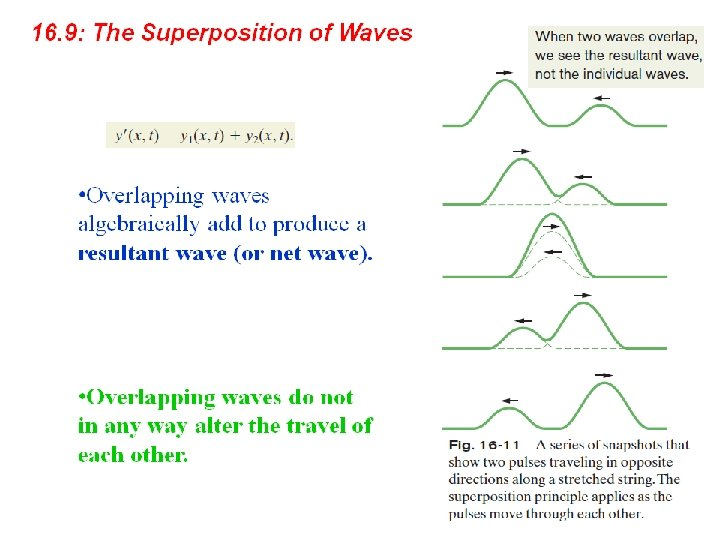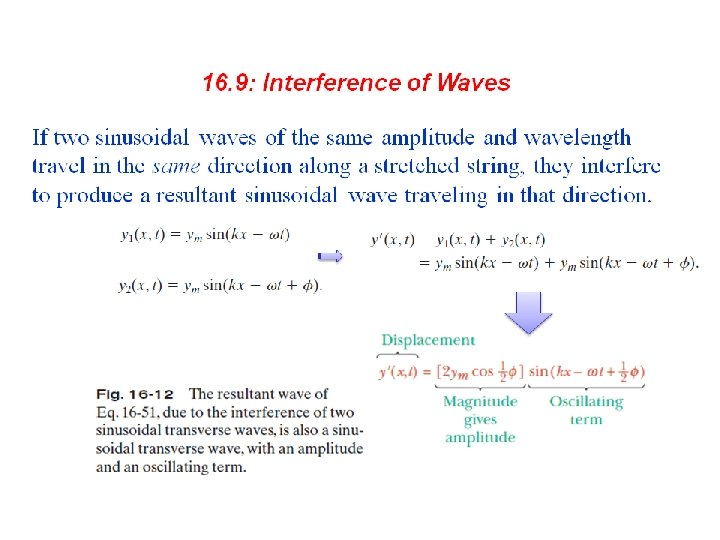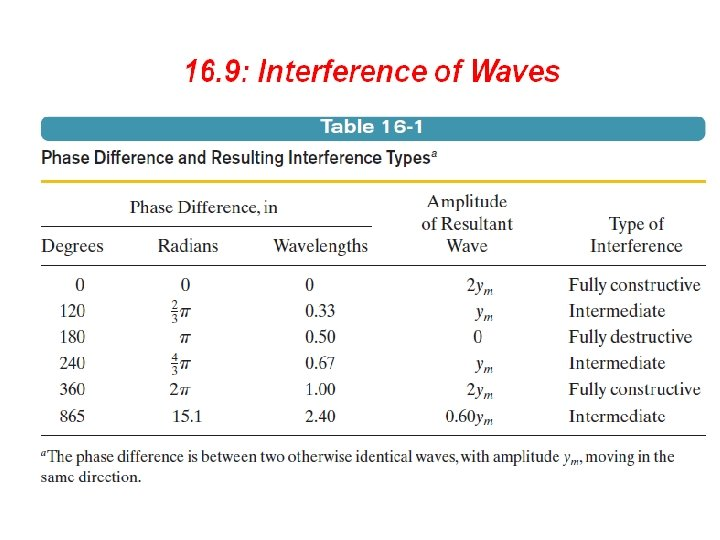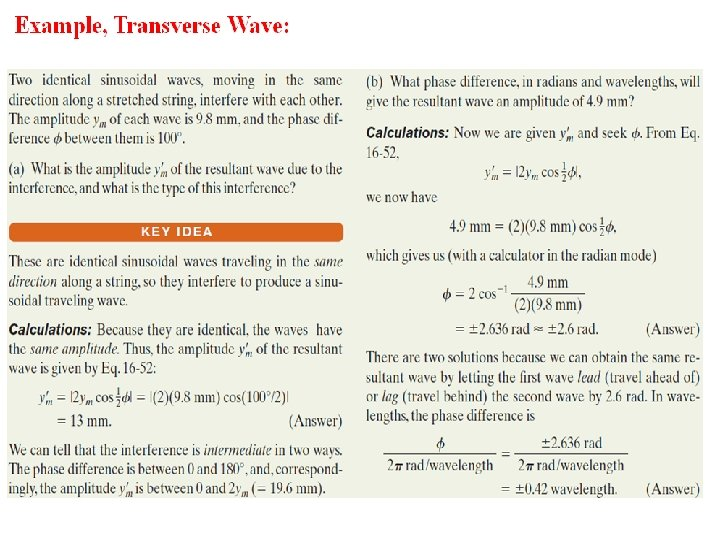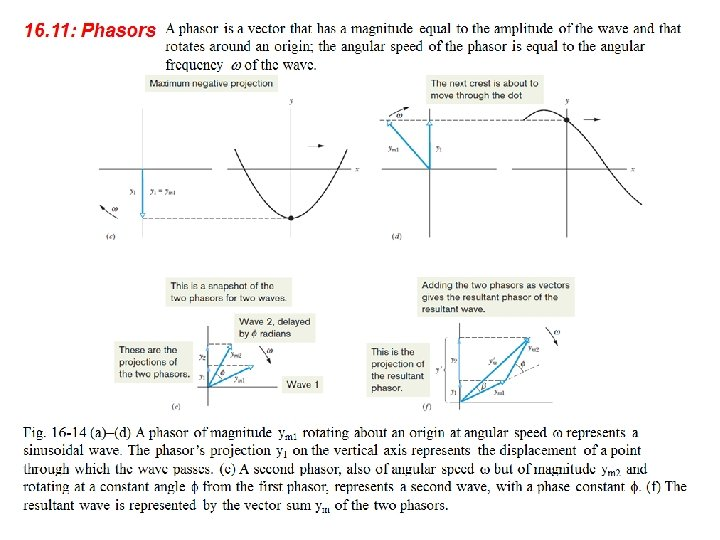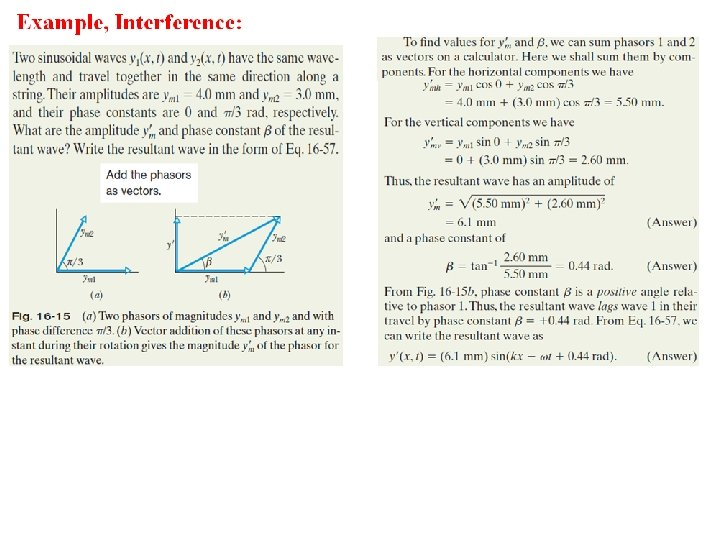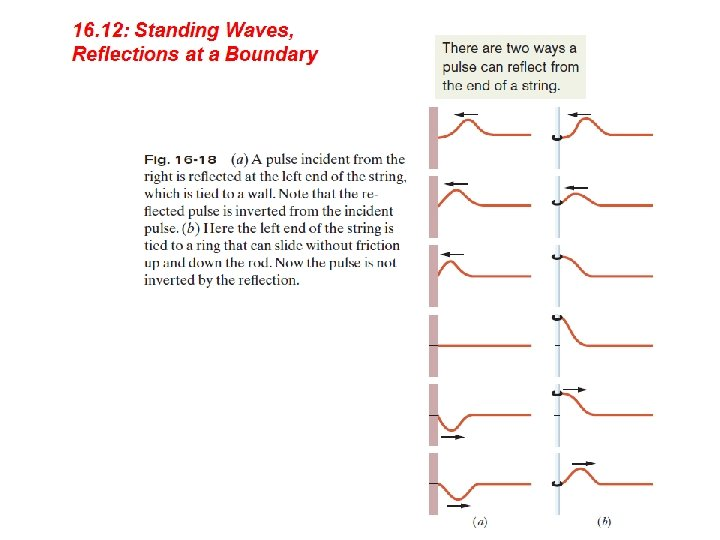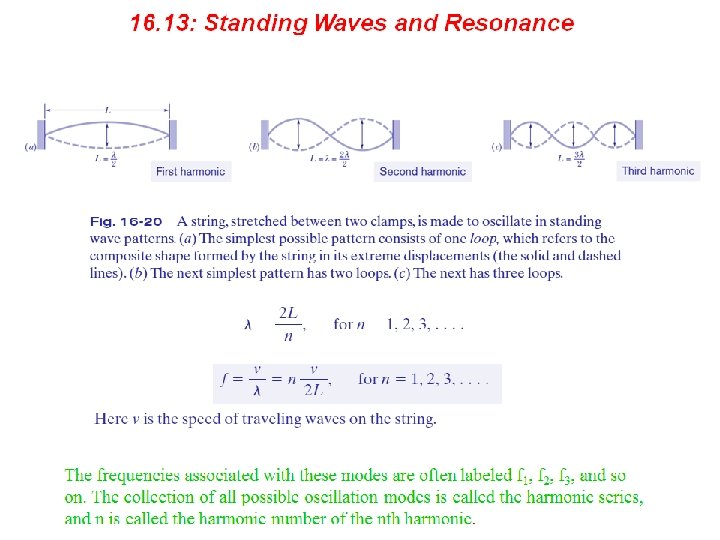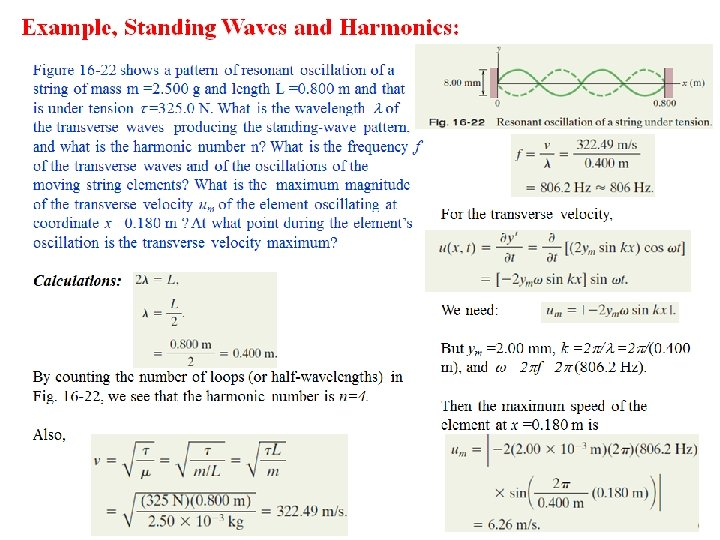• Suggested problems: Chapter 16 • • The quiz questions will be same or very similar to the following text-book problems. Refer to the course website for the latest version of this document. You are encouraged to seek the help of your instructor during his office hours. 5. A sinusoidal wave travels along a string. The time for a particular point to move from maximum displacement to zero is 0. 170 s. What are the (a) period and (b) frequency? (c) The wavelength is 1. 40 m; what is the wave speed? Answer: (a) 0. 680 s; (b) 1. 47 Hz; (c) 2. 06 m/s 12. A The function y(x, t) = (15. 0 cm) cos (πx – 15. 0 πt), with x in meters and t in seconds, describes a wave on a taut string. What is the transverse speed for a point on the string at an instant when that point has the displacement y = +12. 0 cm? Answer: 424 cm/s = 4. 24 m/s 16. The speed of a transverse wave on a string is 170 m/s when the string tension is 120 N. To what value must the tension be changed to raise the wave speed to 180 m/s? Answer: 135 N 32. What phase difference between two identical traveling waves, moving in the same direction along a stretched string, results in the combined wave having an amplitude 1. 50 times that of the common amplitude of the two combining waves? Express your answer in (a) degrees, (b) radians, and (c) wavelengths. Answer: (a) 82. 8 º; (b) 1. 45 rad; (c) 0. 230 λ 49. A nylon guitar string has a linear density of 7. 20 g/m and is under a tension of 150 N. The fixed supports are distance D = 90. 0 cm apart. The string is oscillating in the standing wave pattern shown in Fig. 16 -38. Calculate the (a) speed, (b) wavelength, and (c) frequency of the traveling waves whose superposition gives this standing wave. Answer: (a) 144 m/s; (b) 60. 0 cm; (c) 241 Hz 52. A rope, under a tension of 200 N and fixed at both ends, oscillates in a second-harmonic standing wave pattern. The displacement of the rope is given by y = (0. 10 m) sin (π x/2) sin(12π t), where x = 0 at one end of the rope, x is in meters, and t is in seconds. What are (a) the length of the rope, (b) the speed of the waves on the rope, and (c) the mass of the rope? (d) If the rope oscillates in a third-harmonic standing wave pattern, what will be the period of oscillation? Answer: (a) 4. 0 m; (b) 24 m/s; (c) 1. 4 kg; (d) 0. 11 s 76. A standing wave results from the sum of two transverse traveling waves given by y 1 = 0. 050 cos (πx – 4πt) and y 2 = 0. 050 cos (πx + 4πt), where x, y 1, and y 2 are in meters and t is in seconds. (a) What is the smallest positive value of x that corresponds to a node? Beginning at t = 0, what is the value of the (b) first, (c) second, and (d) third time the particle at x = 0 has zero velocity? Answer: (a) 0. 50 m; (b) 0; (c) 0. 25 s; (d) 0. 50 s • • • • • • 38